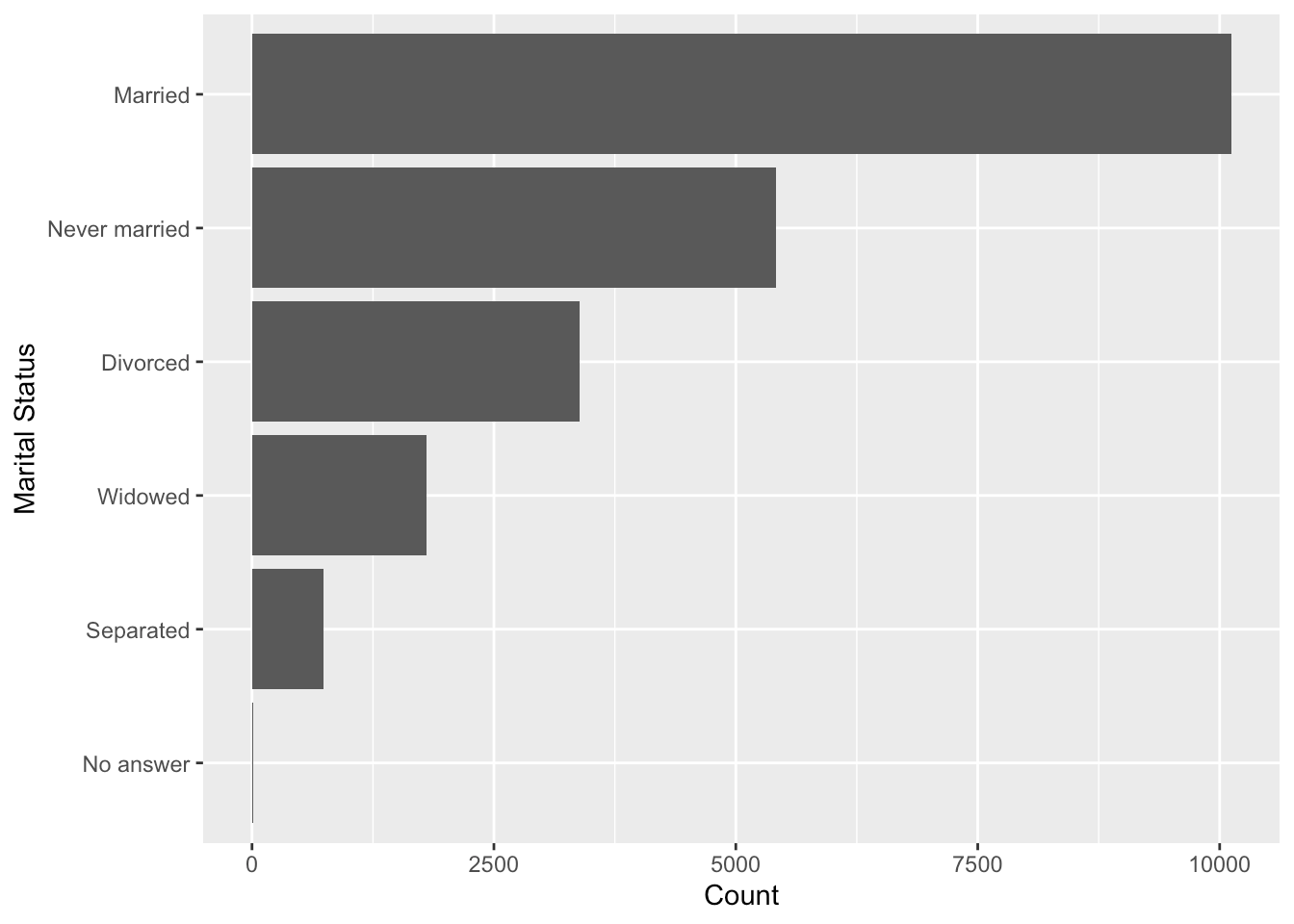Want to share your content on R-bloggers? click here if you have a blog, or here if you don't.

The `magrittr` pipe (`%>%`) has revolutionised the way many people now write R code. I’ve been using R for over 7 years and the pipe has become a staple of my programming conventions. However it was recently brought to my attention that you can actually use pipes within function calls, which can make my code even more human readable. Take for example the following code.

``````library(forcats)
library(dplyr)
gss_cat2 <-
gss_cat %>%
mutate(marital2 = fct_rev(fct_infreq(marital)))``````

Whilst this code is fairly easy to read, you can imagine how this doesn’t scale very nicely with even more nested function calls. One solution would be to use `dplyr`’s ability to `mutate` the same column within the `mutate` function call to split out the code a little more.

``````gss_cat3 <-
gss_cat %>%
mutate(
marital2 = fct_infreq(marital),
marital2 = fct_rev(marital2)
)``````

Sure this is more readable, but it’s repeating far too much code for my liking. So, we can instead just use the pipe.

``````gss_cat4 <-
gss_cat %>%
mutate(
marital2 =
marital %>%
fct_infreq() %>%
fct_rev()
)``````

This not only eliminates long, nested function calls but it also makes my code much more human readable! Try it yourself.

``````ggplot(gss_cat4) +
geom_bar(aes(x = marital2)) +
labs(x = "Marital Status", y = "Count") +
coord_flip()``````